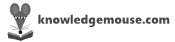# Free Online Printable Geometry Terms Quiz

Created by pandali

This flash card set contains some simple terms from geometry. You can give the definition (like "triangle with two equal sides") and see if the student can come up with the term ("isoceles") or vice versa.

Instructions: Each row makes one card. Cut along the double lines and fold along the single lines. Then glue or tape the sides together.

 Triangle with two equal sides isoceles Quadrilateral with all equal sides square Quadrilateral with two pairs of parallel sidesparallelogram
 90-degree angle right angle Polygon with eight sides octagon 3D object with all points on the surface the same distance from the center sphere Triangle with all equal sides equilateral
 Cut an angle into two equal halves bisect Figure like an elongated circle ellipse 3D object with eight faces octahedron

#### Quick Feedback for Knowledge Mouse

Want to suggest a feature? Report a problem? Suggest a correction? Please let Knowledge Mouse know below: